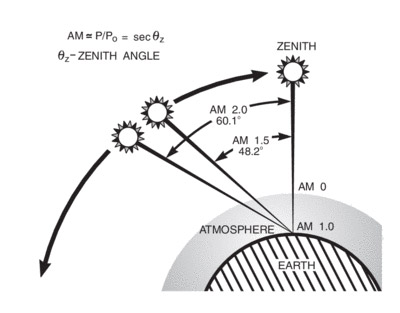A# airmassThe path length in units of air mass, changes with the zenith angle.

Airmass is the path length that light from a celestial object takes through Earth's atmosphere relative to the length at the zenith. Airmass is 1 at the zenith and roughly 2 at an altitude of 60°. It can be calculated to a good approximation using the formula

A = 1.0 / [ cos(Z) + 0.50572 × (96.07995 – Z)–1.6364]

where Z is the zenith angle (the vertical angle of an object from the zenith). This formula comes from Kasten, F. and A. T. Young (1989). "Revised optical air mass tables and approximation formula." Applied Optics 28 (22), 4735–4738.